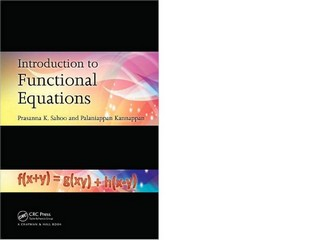### Book Toankho

Sách hay - Sách đẹp

# Introduction to Functional EquationsIntroduction to Functional Equations grew out of a set of class notes from an introductory graduate level course at the University of Louisville. This introductory text communicates an elementary exposition of valued functional equations where the unknown functions take on real or complex values.
In order to make the presentation as manageable as possible for students from a variety of disciplines, the book chooses not to focus on functional equations where the unknown functions take on values on algebraic structures such as groups, rings, or fields. However, each chapter includes sections highlighting various developments of the main equations treated in that chapter. For advanced students, the book introduces functional equations in abstract domains like semigroups, groups, and Banach spaces.

Functional equations covered include:

Cauchy Functional Equations and Applications
The Jensen Functional Equation
Pexider’s Functional Equation
D’Alembert Functional Equation
Trigonometric Functional Equations
Pompeiu Functional Equation
Hosszu Functional Equation
Davison Functional Equation
Abel Functional Equation
Mean Value Type Functional Equations
Functional Equations for Distance Measures
The innovation of solving functional equations lies in finding the right tricks for a particular equation. Accessible and rooted in current theory, methods, and research, this book sharpens mathematical competency and prepares students of mathematics and engineering for further work in advanced functional equations.